# Evolutionary Rates & Selection Strength

#### 2022-05-12

This vignette explains how to extract evolutionary rate parameters estimated from relaxed clock Bayesian inference analyses produced by the program Mr. Bayes. It also shows how to use evolutionary rate based inference of selection strength (or mode) adapted to clock-based rates, as introduced by .

## Evolutionary Rates Statistics and Plots

In this section, we will extract evolutionary rate parameters from each node from a Bayesian clock (time-calibrate) summary tree produced by Mr. Bayes. The functions below will store them in a data frame, produce summary statistics tables, and create different plots showing how rates are distributed across morphological partitions and clades.

library(EvoPhylo)

### 1. Get rates from the clock tree and create a rate table

First, import a Bayesian clock tree using treeio’s function read.mrbayes() (= read.beast()).

## Import summary tree with three clock partitions produced by
## Mr. Bayes (.t or .tre files) from your local directory
tree3p <- treeio::read.mrbayes("Tree3p.t")

Below, we use the example tree tree3p that accompanies EvoPhylo.

data(tree3p)

Subsequently, using get_clockrate_table(), users can extract mean or median rate values for each node in the summary tree that were annotated by Mr. Bayes when creating the summary tree with Mr. Bayes “sumt” command. These mean or median rate values are calculated by Mr. Bayes taking into account all trees from the posterior sample. This works for any summary tree produced by Mr. Bayes: a majority rule consensus or the fully resolved maximum compatible tree (the latter is used in the examples here).

Please note that analyses must have reached the stationarity phase and independent runs converging for the summary statistics in each node to be meaningful summaries of the posterior sample.

## Get table of clock rates with summary stats for each node in
## the tree for each relaxed clock partition
rate_table_means_no_clades3 <- get_clockrate_table(tree3p, summary = "mean")

### 2. Export the rate table

This is a necessary step to subsequently open the rate table spreadsheet locally (e.g., using Microsoft Office Excel) and customize the table with clade names associated with with each node in the tree for downstream analysis.

## Export the rate tables
write.csv(rate_table_means_no_clades3, file = "RateTable_Means3.csv")

### 3. Plot tree node labels

To visualize the node values in the tree, you can use ggtree().

## Plot tree node labels
library(ggtree)
tree_nodes <- ggtree(tree3p, branch.length = "none", size = 0.05) +
geom_tiplab(size = 2, linesize = 0.01, color = "black", offset = 0.5) +
geom_label(aes(label = node), size = 2, color="purple", position = "dodge")
tree_nodes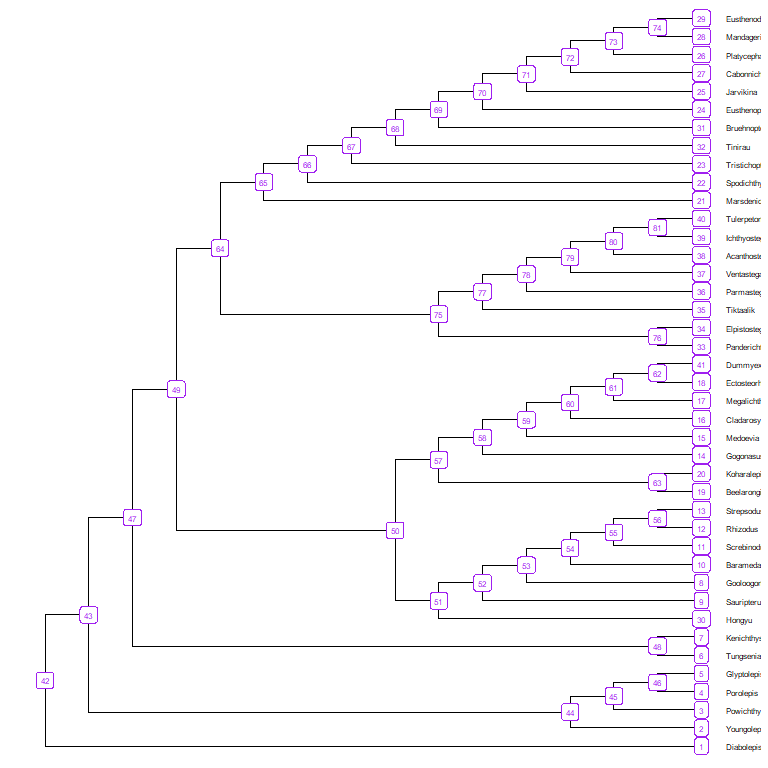## Save your plot to your working directory as a PDF
ggplot2::ggsave("Tree_nodes.pdf", width = 10, height = 10)

### 4. Get summary statistics table and plots

## Import rate table with clade membership (new "clade" column added)
rate_table_clades_means3 <- read.csv("RateTable_Means3_Clades.csv", header = TRUE)

Below, we use the rate table with clade membership rate_table_clades_means3 that accompanies EvoPhylo.

data(rate_table_clades_means3)

head(rate_table_clades_means3)
##         clade nodes   rates1   rates2   rates3
## 1 Dipnomorpha     1 0.943696 0.981486 1.006164
## 2 Dipnomorpha     2 1.065326 0.772074 0.913194
## 3 Dipnomorpha     3 1.182460 0.656872 0.813618
## 4 Dipnomorpha     4 1.229767 0.523709 0.722519
## 5 Dipnomorpha     5 1.230564 0.517773 0.720479
## 6       Other     6 0.658855 0.717277 0.663950

Obtain summary statistics table and plots for each clade by clock using clockrate_summary(). Supplying a file path to file save the output to that file.

## Get summary statistics table for each clade by clock
file = "Sum_RateTable_Means3.csv")
Rate table summary statistics
clade clock n mean sd min Q1 median Q3 max
Dipnomorpha 1 8 1.10 0.11 0.94 1.02 1.10 1.19 1.23
Elpisostegalia 1 14 1.61 0.22 1.13 1.45 1.68 1.80 1.81
Osteolepididae 1 11 0.63 0.26 0.16 0.44 0.81 0.84 0.87
Rhizodontidae 1 14 0.57 0.30 0.03 0.33 0.67 0.83 0.89
Tristichopteridae 1 21 0.71 0.04 0.61 0.69 0.72 0.74 0.78
Other 1 11 0.89 0.36 0.54 0.69 0.78 0.94 1.81
Dipnomorpha 2 8 0.75 0.18 0.52 0.62 0.75 0.89 0.98
Elpisostegalia 2 14 1.36 0.10 1.03 1.36 1.38 1.41 1.42
Osteolepididae 2 11 0.34 0.15 0.07 0.28 0.38 0.45 0.53
Rhizodontidae 2 14 0.33 0.18 0.02 0.17 0.38 0.44 0.56
Tristichopteridae 2 21 0.34 0.06 0.27 0.32 0.33 0.33 0.55
Other 2 11 0.75 0.25 0.39 0.61 0.72 0.78 1.35
Dipnomorpha 3 8 0.87 0.11 0.72 0.79 0.89 0.95 1.01
Elpisostegalia 3 14 0.83 0.16 0.63 0.67 0.89 0.99 1.00
Osteolepididae 3 11 0.32 0.13 0.07 0.27 0.33 0.42 0.49
Rhizodontidae 3 14 0.32 0.17 0.02 0.21 0.40 0.43 0.52
Tristichopteridae 3 21 0.52 0.08 0.37 0.44 0.54 0.59 0.64
Other 3 11 0.73 0.17 0.47 0.64 0.70 0.81 1.00

### 5. Plot rates by clock partition and clade

Plot distributions of rates by clock partition and clade with clockrate_dens_plot().

## Overlapping plots
nrow = 1, scales = "fixed")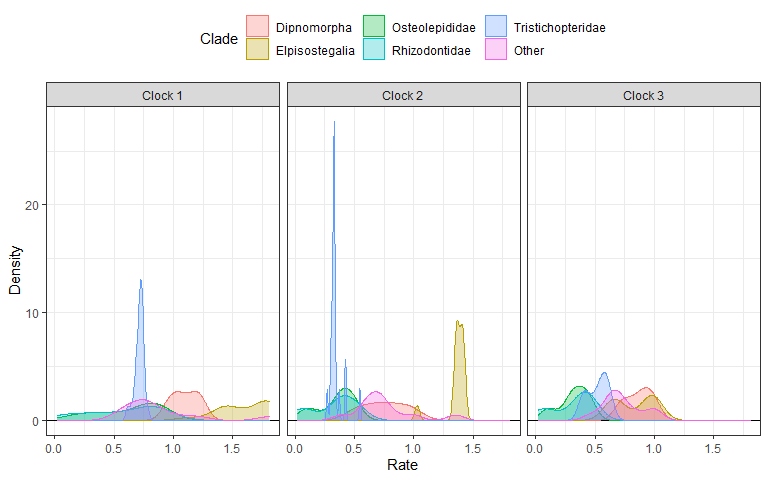Sometimes using stacked plots provides a better visualization as it avoids overlapping distributions.

## Stacked plots
nrow = 1, scales = "fixed")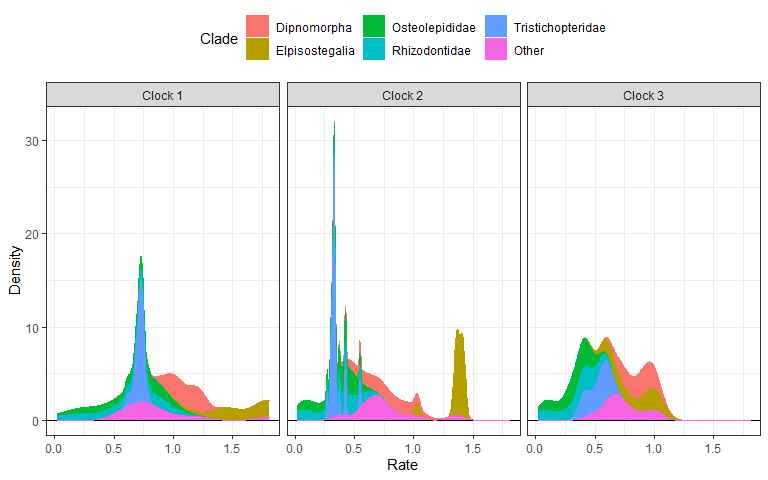It is also possible to append extra layers using ggplot2 function, such as for changing the color scale. Below, we change the color scale to be the Viridis scale.

## Stacked plots with viridis color scale
nrow = 1, scales = "fixed") +
ggplot2::scale_color_viridis_d() +
ggplot2::scale_fill_viridis_d()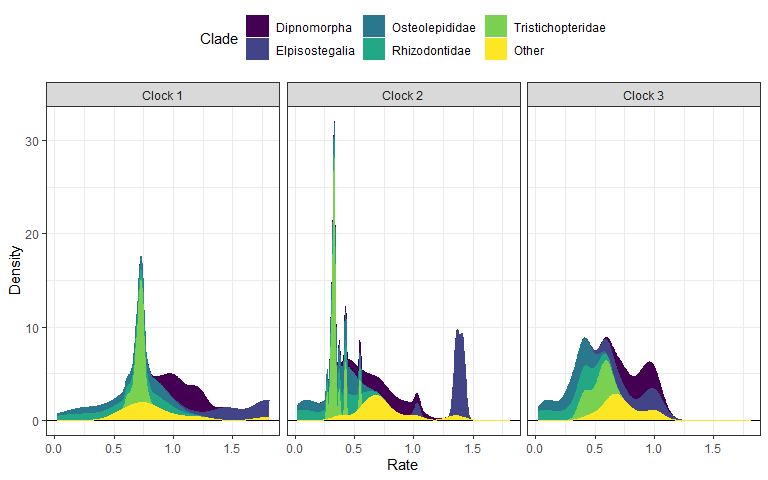### 6. Rate linear models

We can also plot linear model regressions between rates from two or more clocks with clockrate_reg_plot().

## Plot regressions of rates from two clocks
p12 <- clockrate_reg_plot(rate_table_clades_means3, clock_x = 1, clock_y = 2)
p13 <- clockrate_reg_plot(rate_table_clades_means3, clock_x = 1, clock_y = 3)
p23 <- clockrate_reg_plot(rate_table_clades_means3, clock_x = 2, clock_y = 3)

library(patchwork) #for combining plots
p12 + p13 + p23 + plot_layout(ncol = 2)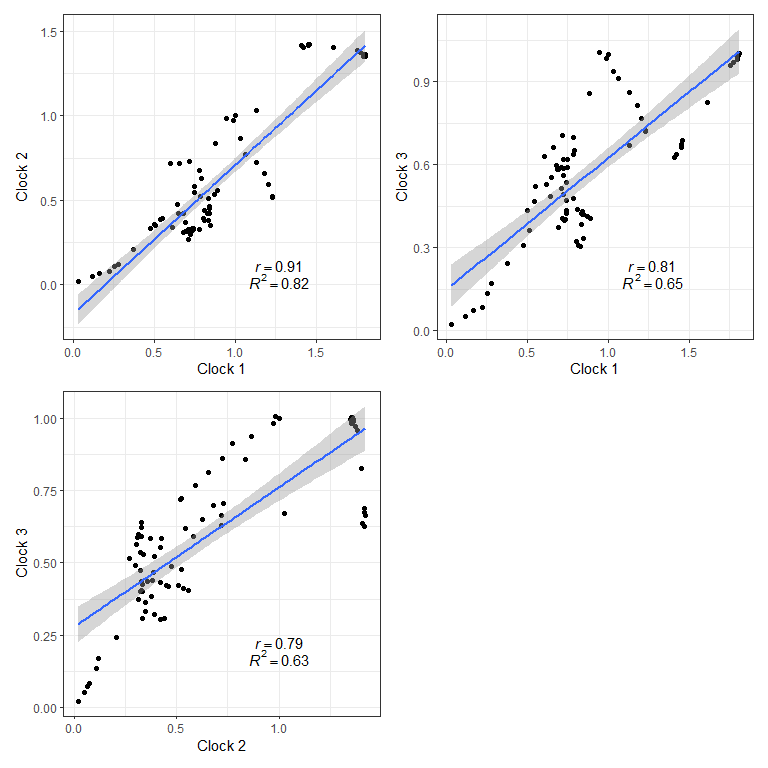## Save your plot to your working directory as a PDF
ggplot2::ggsave("Plot_regs.pdf", width = 8, height = 8)

### Rates from single clock analysis

You can also explore clock rates for summary trees including a single clock shared among all character partitions (or an unpartitioned analysis):

## Import summary tree with a single clock partitions produced by
## Mr. Bayes (.t or .tre files) from examples directory
tree1p <- treeio::read.mrbayes("Tree1p.t")

Below, we use the example tree tree1p that accompanies EvoPhylo.

data(tree1p)

Then, get table of clock rates with summary stats for each node in the tree for each relaxed clock partition.

rate_table_means_no_clades1 <- get_clockrate_table(tree1p, summary = "mean")
## Export the rate tables

rate_table_clades_means1 <- read.csv("RateTable_Means1_Clades.csv", header = TRUE)
data(rate_table_clades_means1)
## Get summary statistics table for each clade by clock
file = "Sum_RateTable_Medians1.csv")
Rate table summary statistics
clade n mean sd min Q1 median Q3 max
Dipnomorpha 8 0.57 0.28 0.22 0.37 0.54 0.78 0.95
Elpisostegalia 14 0.91 0.25 0.44 0.77 0.85 1.03 1.35
Osteolepididae 11 0.23 0.10 0.03 0.18 0.23 0.30 0.38
Rhizodontidae 14 0.18 0.15 0.00 0.04 0.20 0.29 0.42
Tristichopteridae 21 0.39 0.43 0.05 0.11 0.19 0.34 1.32
Other 11 0.41 0.26 0.20 0.25 0.28 0.45 1.00
## Stacked plots with viridis color scale
nrow = 1, scales = "fixed") +
ggplot2::scale_color_viridis_d() +
ggplot2::scale_fill_viridis_d()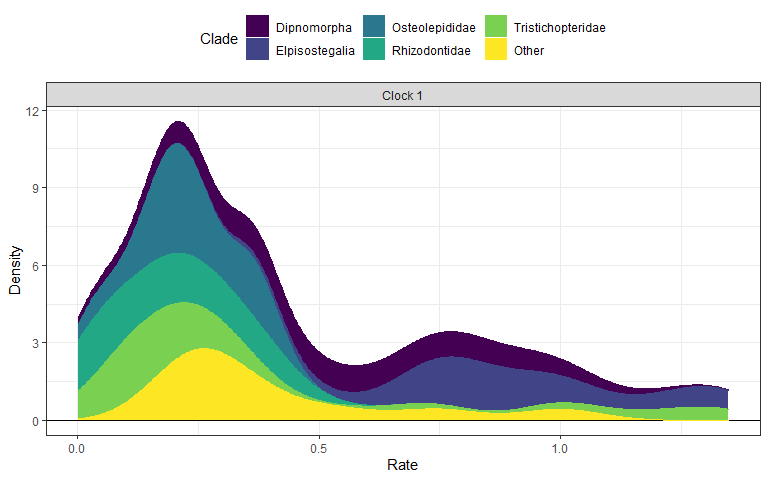## Selection strength (mode)

In this section, we will use evolutionary rate based inference of selection strength (or mode), as first introduced by for continuous traits, and later adapted to clock-based rates by .

### 1. Import and transform table

## Import rate table with clade membership (new "clade" column added)
## from your local directory with "mean" values
rate_table_clades_means3 <- read.csv("RateTable_Means3_Clades.csv", header = TRUE)

Below, we use the rate table with clade membership rate_table_clades_means3 that accompanies EvoPhylo.

data(rate_table_clades_means3)

It is necessary to transform the table from wide to long format with clock_reshape().

## Transform table from wide to long format
rates_by_clade <- clock_reshape(rate_table_clades_means3)

### 2. Import combined log file from all runs.

This is produced by using combine_log(). Alternatively, users can also use LogCombiner from the BEAST2 software package. The first argument passed to combine_log() should be a path to the folder containing the log files to be imported and combined.

## Import all log (.p) files from all runs and combine them, with burn-in = 25%
## and downsampling to 2.5k trees in each log file
posterior3p <- combine_log("LogFiles3p", burnin = 0.25, downsample = 1000)

Below, we use the posterior dataset posterior3p that accompanies EvoPhylo.

data(posterior3p)

## Show first 10 lines of combined log file
head(posterior3p, 10)

### 3. Pairwise t-tests of Rate values

The function get_pwt_rates() will produce a table of pairwise t-tests for differences between the mean clockrate value in the posterior and the absolute rate for each tree node.

## Get table of pairwise t-tests for difference between the posterior
## mean and the rate for each tree node

## Show first 10 lines of table
head(rate_sign_tests, 10)
Combined log file
clade nodes clock relative rate absolute rate (mean) null p.value
Dipnomorpha 1 1 0.943696 0.0118443 0.0118443 0
Dipnomorpha 2 1 1.065326 0.0133709 0.0133709 0
Dipnomorpha 3 1 1.182460 0.0148411 0.0148411 0
Dipnomorpha 4 1 1.229767 0.0154348 0.0154348 0
Dipnomorpha 5 1 1.230564 0.0154448 0.0154448 0
Other 6 1 0.658855 0.0082693 0.0082693 0
Other 7 1 0.603090 0.0075694 0.0075694 0
Osteolepididae 8 1 0.843373 0.0105852 0.0105852 0
Osteolepididae 9 1 0.872012 0.0109446 0.0109446 0
Osteolepididae 10 1 0.811473 0.0101848 0.0101848 0
## Export the table
write.csv(rate_sign_tests, file = "RateSign_tests.csv")

### 4. Plot selection gradient on the summary tree

Using different thresholds, Identify the strength (or mode) across branches in the tree for each clock partition with plot_treerates_sgn().

## Plot tree using various thresholds for clock partition 1
A1 <- plot_treerates_sgn(
tree3p, posterior3p,
clock = 1,               #Show rates for clock partition 1
summary = "mean",        #sets summary stats to get from summary tree nodes
branch_size = 1.5, tip_size = 3,                      #sets size for tree elements
xlim = c(-450, -260), nbreaks = 8, geo_size = list(3, 3),  #sets limits and breaks for geoscale
threshold = c("1 SD", "2 SD"))                 #sets threshold for selection mode
A1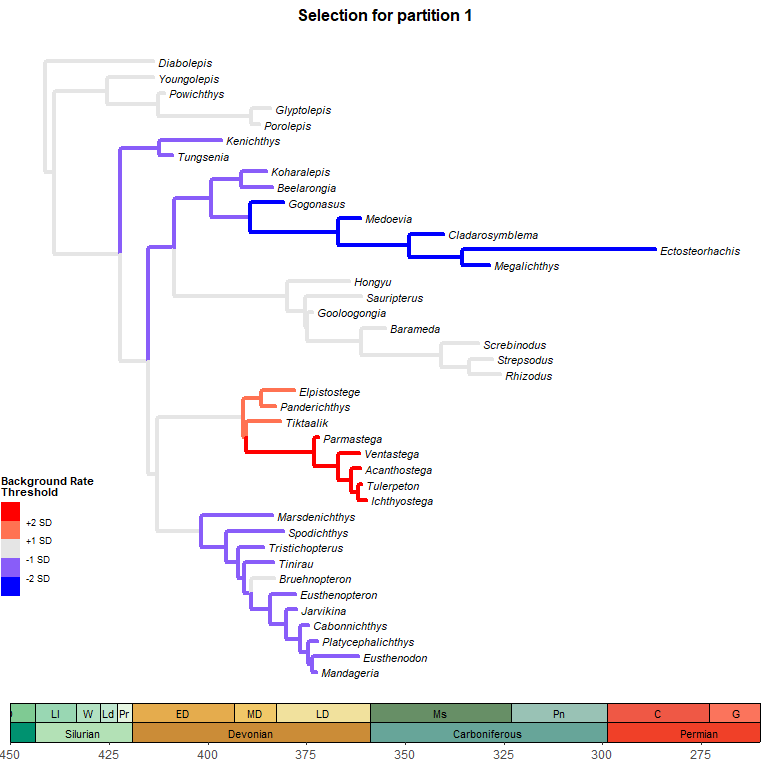Plot tree using various thresholds for the other clock partitions and combine them.

## Plot tree using various thresholds for other clock partition and combine them
A2 <- plot_treerates_sgn(
tree3p, posterior3p,
clock = 2,               #Show rates for clock partition 2
summary = "mean",        #sets summary stats to get from summary tree nodes
branch_size = 1.5, tip_size = 3,                      #sets size for tree elements
xlim = c(-450, -260), nbreaks = 8, geo_size = list(3, 3),  #sets limits and breaks for geoscale
threshold = c("1 SD", "2 SD"))                 #sets threshold for selection mode

A3 <- plot_treerates_sgn(
tree3p, posterior3p,
clock = 3,               #Show rates for clock partition 2
summary = "mean",        #sets summary stats to get from summary tree nodes
branch_size = 1.5, tip_size = 3,                      #sets size for tree elements
xlim = c(-450, -260), nbreaks = 8, geo_size = list(3, 3),  #sets limits and breaks for geoscale
threshold = c("1 SD", "2 SD"))                 #sets threshold for selection mode

library(patchwork)
A1 + A2 + A3 + plot_layout(nrow = 1)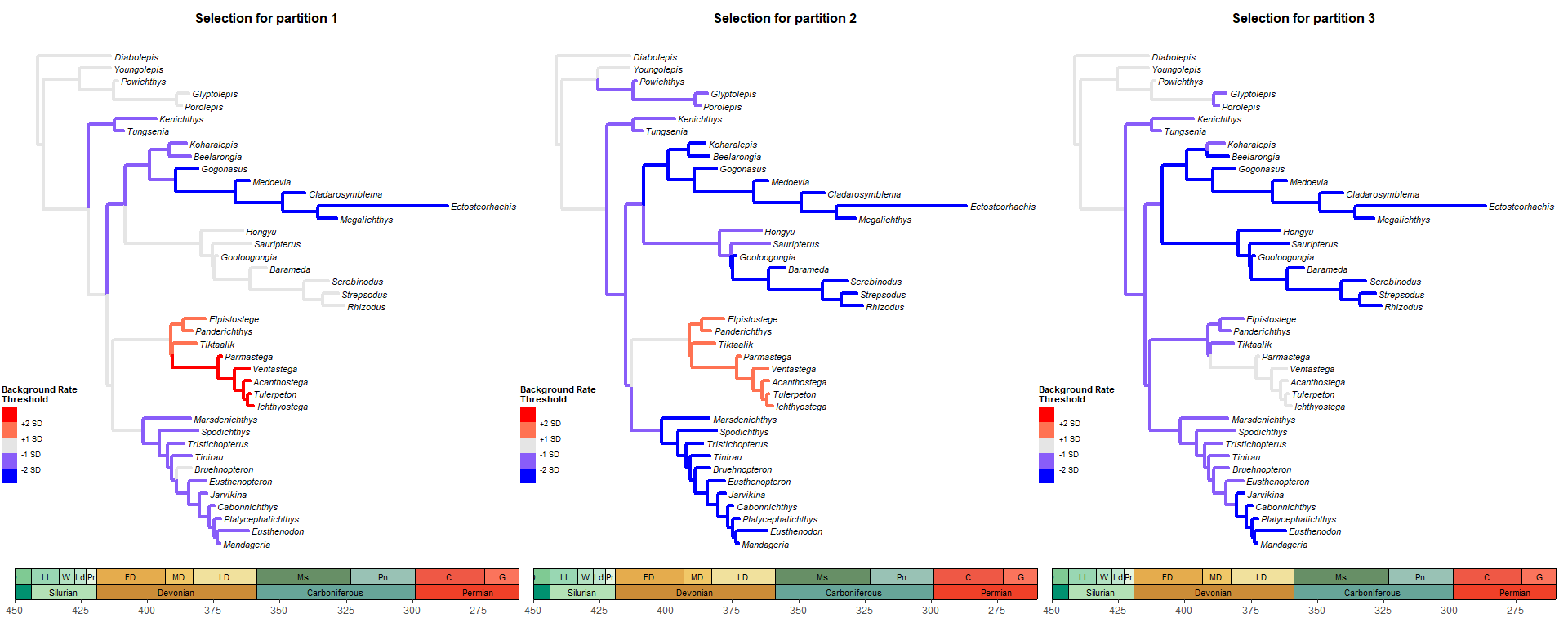## Save your plot to your working directory as a PDF
ggplot2::ggsave("Tree_Sel_3p.pdf", width = 20, height = 8)